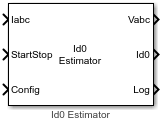# Id0 Estimator

Estimate magnetizing current (Id0) of AC induction motor (ACIM)

Since R2023a

•Libraries:
Motor Control Blockset / Parameter Estimation / ACIM Parameter Estimation

## Description

The Id0 Estimator block computes the magnetizing current of an induction motor using the phase currents and the test configuration elements.

The block runs the induction motor using open-loop control, during which it measures the peak current response values and averages them out over a given period. In addition, the block measures the time taken by the motor to reach from zero to 90 percent of the rated frequency. It uses this time duration as well as the computed average current to estimate the magnetizing current of the induction motor.

## Ports

### Input

expand all

The three-phase current response of the motor measured by the current sensors.

Note

Providing phase-c current input is optional. The block functionality is not impacted if you do not provide the phase-c current input.

Data Types: `single`

Signal that either starts or stops Id0 estimation:

• `1` (rising edge of a pulse) — This signal initiates Id0 estimation.

• `0` (falling edge of a pulse) — This signal immediately stops the Id0 estimation process. If you interrupt the estimation process abruptly before completion, the block does not compute or output the Id0 value.

Data Types: `Boolean`

This table summarizes the expected configuration elements in the bus signal input.

CategoryConfiguration elementUnits

Elements for motor and inverter hardware

Number of pole pairs

-

Rated frequency

Hz

Nominal motor current

A

Nominal voltage

V

Maximum measurable inverter current

A

Board resistance

ohm

Current sense gain

Voltage sense gain

Elements for estimating magnetizing current Id0

Measurement time

seconds

Open-loop ramp time

seconds

Elements for estimating motor resistance (Rs)

Estimation time

seconds

Vd reference voltage 1

PU voltage

Vd reference voltage 2

PU voltage

Elements for estimating rotor resistance (Rr), magnetizing inductance (Lm), stator leakage inductance (Lls), and rotor leakage inductance (Llr)

Frequency 1 as a ratio of rated frequency

PU frequency

Frequency 2 as a ratio of rated frequency

PU frequency

High frequency for Lm

Hz

Start voltage for voltage sweep

PU voltage

Voltage step for voltage sweep

PU voltage

High-frequency injection voltage

PU voltage

Elements for estimating motor inertia (J) and friction constant (B)

Closed-loop speed duration

seconds

Closed-loop speed

RPM

Iq Reference current for FOC

PU current

Proportional gain

-

Integral gain

-

Lower speed limit for inertia estimation

RPM

-

Parameter estimation sample time

seconds

You can connect this input port to the `Config` output port of the ACIM Parameter Estimation Configurator block.

Data Types: `single`

### Output

expand all

Stator reference voltage components along the a, b, and c axes of the abc reference frame.

Data Types: `single`

Estimated magnetizing current (in Amperes).

Data Types: `single`

Debug signals that the block provides for examination. The bus includes these debug signals.

Debug signalDescriptionUnits

Id

D-axis current

Amperes

status

`0`Did not complete Id0

-

`1`Id0 estimation completed successfully

-

Data Types: `single`

## Version History

Introduced in R2023a